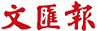首頁 > 文匯報 > 教育 > 正文

# 【奧數揭秘】計算日期說「同餘」

2017-03-0817n≡10n(mod7)

≡3n(mod7)

2300=(23)100

=8100

=1100(mod7)

=1

194+282=131104

194+282≡14+12(mod9)

=2

131104≡1+3+1+1+0+4(mod9)

=10

=1